# Approximating Formulas for the USCF Rating System

This document provides approximation formulas for players to compute and update their USCF ratings. The actual rating system is quite complex, and performing the computations by hand is virtually impossible. It should be noted that, on occasion, the formulas presented below produce ratings considerably different from ratings calculated under the actual algorithm, so that the formulas below should serve only as a rough guide. A detailed description of the new system's formulas is posted at the USCF web site (www.uschess.org), and is available on request from the USCF.

Provisional and established ratings:

Players' ratings are considered provisional if they have played 25 or fewer games (rather than 20 under the old system), and established if having played more than 25. There are two different formulas to compute ratings. The criterion for using the different formulas depends on whether the player has completed 8 tournament games. The formula for ratings based on 8 or fewer games is called the special'' rating formula, and the other is called the standard'' formula. A provisional rating is updated using the special formula if the number of completed games is 8 or fewer, and the standard formula if the number is greater than 8. Established ratings are based on the standard formula.

Special rating formula:

If a player has a rating based on 8 or fewer games, or is unrated, then the new rating can be approximated by the old provisional rating formula, that is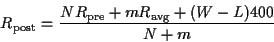where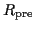is the player's pre-tournament rating,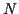is the number of games upon whichis based,is the number of games the player completes in the tournament,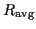is the average of the opponents' ratings,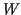is the number of wins, and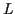is the number of losses. If the player is unrated, set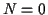and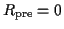. The final result is rounded to an integer.

Example: Suppose a player rated 1500 based on 6 games competes against players rated 1400, 1550 and 1650, winning the first, losing the second and drawing the third. In this case,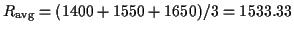,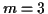,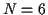,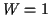,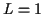, and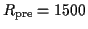. Then, from the special rating formula,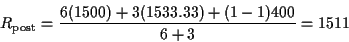The final result has been rounded from 1511.111.

It should be noted that, from the approximating formula, a player could gain rating points by losing to a high rated player, or lose rating points with a win over a low rated player. The actual rating procedure corrects for these possibilities. Furthermore, the actual formulas first calculate ratings for unrated opponents, thereby making use of all game outcomes.

Standard rating formula:

To approximate one's rating using the standard formulas, a player needs to know (or approximate) the number of games played in tournaments, only if less than 50. Letbe the number of previous games, but setto 50 if the number of games is 50 or more. Then, if the player has a pre-tournament rating less than then 2200, the player computes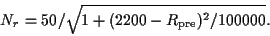If the player's rating is 2200 or greater, then set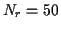. Finally, let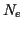be the smaller ofand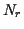. This number, the effective'' number of games upon which a rating is based, can be calculated before entering a tournament.

Example: Suppose a player's pre-tournament rating is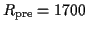, based on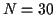games. Then, according to the formula above,Because 26.7 is smaller than 30,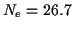is the effective number of games for this player.

The next step in the calculation is to determine the value of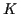, the value that governs the magnitude of rating changes. For a full-event, lettingbe the number of games the players completes in the tournament,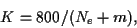and for half-,Finally, oncehas been computed, the formula for updating a player's rating is given by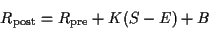whereis the pre-tournament rating,is the value just computed,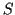is the total score in the tournament (counting 1 for each win, 0.5 for each draw and 0 for each loss),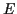is the sum of winning expectancies (described below), and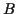is a possible bonus amount (described below). The final value is rounded to the nearest integer. In the actual formulas, the rating is rounded away from the pre-event rating.

To calculate, the winning expectancy for each opponent must be calculated and then summed. The formula for the winning expectancy between a player with ratingand an opponent with rating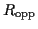is given by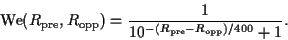This is computed for each opponent, and the results are totaled to produce a value of.

The bonus,, is automatically 0 if the player has competed against fewer than 3 distinct opponents, or more than twice against any opponent. If the player has competed against 3 or more opponents, no more than twice against each, then a comparison is made between the value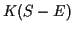and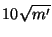, where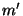is the larger ofand 4 (in other words, 3-round events are treated as 4-round events when computing the bonus amount). Ifis larger or equal, then the bonus is 0. But ifis larger, then the bonus is the difference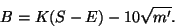This is the extra amount added to one's rating for having an unusually strong result. Please note that the value 10'' in the formula will revert to 16'' in January 2003, resulting in greater difficulty to obtain bonus amounts.

Example: Suppose a player is rated 1300 based on 45 games, and competes in a full-event against 4 distinct opponents rated 1250, 1400, 1500, and 1550 winning three and drawing one. With these results,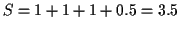.

First, we compute the value ofas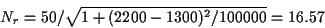so that the lower of 16.57 and 45 is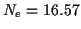.

The value offor this player in this tournament is given by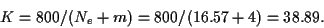The winning expectancy against the opponent rated 1250 is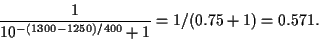Similarly, the winning expectancies against the other three opponents are computed as 0.360, 0.240 and 0.192. Adding these four values results in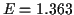. Finally, becauseis larger than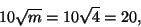the bonus is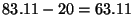. The final approximated rating is therefore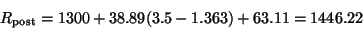which is then rounded up to 1447.

Rating floors:

Rating floors exist at 100, 1400, 1500, 1600,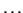, 2100. No player's rating can drop below 100. A player's rating floor is calculated by subtracting 200 points from the highest attained established rating, and then using the floor just below. For example, if a player's highest rating was 1941, then subtracting 200 yields 1741, and the floor just below is 1700. Thus the player's rating cannot go below 1700. If a player's highest rating was 1588, then subtracting 200 yields 1388, and the next lowest floor is 100, which is this player's floor.